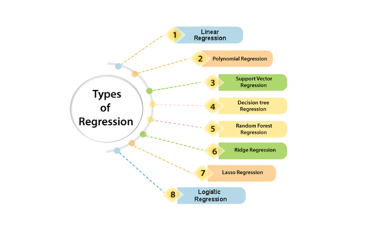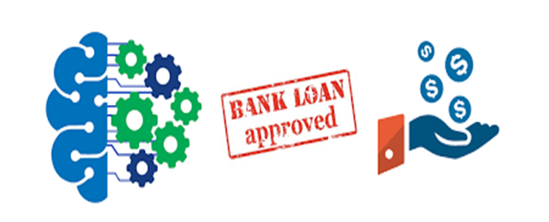Binary Logistic Regression Interpretation

# Binary Logistic Regression Interpretation

2305

Logistic regression is a type of regression analysis. So, before we delve into logistic regression, let us first understand the general concept of regression analysis.

## What is Regression?

A type of predictive modeling technique which is used to find the relationship between a dependent variable, Y and either one independent variable, X or a series of independent variables is what we mean by the term regression analysis. Regression analysis can be broadly classified into different types: Linear, Polynomial, Support Vector, Decision Tree, Random Forest, Ridge, Lasso and Logistic Regression.## Brief description on Logistic Regression

Logistic regression is essentially used to predict the probability of a binary (yes/no) event occurring. It is more robust than linear regression to outliers in the data. It is considered to be the proper regression analysis to implement when the dependent variable is binary i.e., dichotomous. Logistic regression can be categorized into binomial, ordinal or multinomial.

Binomial or binary logistic regression is impacted with conditions where the observed outcome for the dependent variable is having only two possible labels, either "0" and "1" (which may represent, for example, "negative" vs. "positive").

Multinomial logistic regression deals with circumstances where the outcome is having three or more possible types (e.g., "negative" vs. "positive" vs. “neutral") and which are not ordered.

Ordinal logistic regression particularly deals with ordered dependent variables.

In this particular article, we will focus mainly on binary logistic regression and try to understand its technical concept to as much depth as possible.

## Binary Logistic Regression

Technical Definition

In binary logistic regression, the outcome is usually labelled as "0" or "1", as this leads to the most straightforward interpretation. This technique helps to identify important factors (Xi) impacting the target variable (Y) and also the nature of the relationship between each of these factors and the dependent variable.

If a particular observed outcome for the dependent variable is the noteworthy possible outcome (referred to as a "success" or an "instance" or a "case") it is usually coded as "1" and on the contrary, the outcome (referred to as a "failure" or a "noninstance" or a "non case") as "0". Binary logistic regression is implemented to predict the odds of a case based on the values of the independent variables (predictors). The odds are defined as the probability that a particular outcome is a case divided by the probability that it is a non-instance.

Types of questions Binary Logistic Regression can answer

Binary Logistic Regression is useful in the analysis of multiple factors influencing a negative/positive outcome, or any other classification where there are only two possible outcomes.

Let’s look at a few use cases where Binary Logistic Regression Classification might be applied and how it would be useful to the organization.

Use Case – 1

Business Problem: A bank loans officer wants to predict if loan applicants will be a bank defaulter or non-defaulter based on various attributes. Here the target variable would be ‘past default status’ and predicted class would include values ‘yes or no’ representing ‘likely to default/unlikely to default’ class respectively.Use Case – 2

Business Problem: A doctor wants to predict the likelihood of a successful treatment of a new patient condition based on various attributes of a patient. Here the target variable would be ‘past cure status’ and the predicted class would contain values ‘yes or no’ meaning ‘prone to cure/not prone to cure’ respectively.

Use Case – 3

Prediction of Lung Cancer: The probability of getting lung cancer (yes vs. no) change for every person depending on every pack of cigarettes smoked per day?

Use Case - 4

Prediction of Heart Attack: The probability of having a heart attack (yes vs. no) is dependent clearly on body weight, calorie intake, fat intake, and age

Binary Logistic Regression major assumptions

1. The dependent variable should be dichotomous in nature (e.g., presence vs. absence).
2. Outliers should not be present in the data, which can be assessed by converting the continuous predictors to standardized scores, and removing values below -3.29 or greater than 3.29.
3. The foremost and most vital task of logistic regression analysis is estimating the log odds of an event.

Parameters in Binary Logistic Regression

For the model to be a cent percent accurate one, we need to calculate and find out a few parameters of the algorithm in order to check how accurate our Binary Logistic Regression model is. The key parameters we calculate and check are dependent on the topic called Confusion Matrix.

What is the Confusion Matrix?

The confusion matrix is a type of table used to define the characteristics of Classification problems. The below are few expressions calculated in order to find how accurate the prediction of the model is.

Accuracy Recall Precision F1 scoreHighlights of Binary Logistic Regression

1. It performs well when the dataset is linearly separable.
2. Normally, it is less prone to overfitting but it can overfit in high dimensional datasets. In such cases, we should consider Regularization (L1 and L2) techniques to avoid over-fitting in these scenarios.
3. This regression not only gives a measure of how relevant a predictor is, but also its direction of association (positive or negative).
4. Moreover, it is easier to implement, interpret and very efficient to train.

Drawbacks of Binary Logistic Regression

1. Main limitation lies in the assumption of linearity between the dependent variable and the independent variables. In the real scenario, the data present is rarely and linearly separable. Most of the time it would be a jumbled mess.
2. If the number of observations are lesser than the number of features, then this regression is not suitable for implementing, otherwise it may lead to overfit.
3. As it can only be used to predict discrete functions. Therefore, the dependent variable is restricted to the discrete number set. This restriction itself seems to be problematic, as it is prohibitive to the prediction of continuous flow of data.Final thoughts

So there we have it: A complete and depth understanding of binary logistic regression. Let’s have a few takeaways to summarize what we’ve covered: There are different types of regression analysis which are already discussed above. Consequently, Logistic regression also has three types. It is important to choose the right model of regression based on the dependent and independent variables of your data. Logistic regression is used for problems where the main objective is classification and accordingly, the output or dependent variable is dichotomous or categorical. Logistic regression can be categorized into binomial, ordinal or multinomial. The technical idea of binary logistic regression in layman’s explanation and its various use cases in real time applications. There are some key assumptions which should be kept in mind while implementing binary logistic regressions. Parameters for an error-free prediction using this regression in our models. The pros and cons Hopefully this post has been useful!